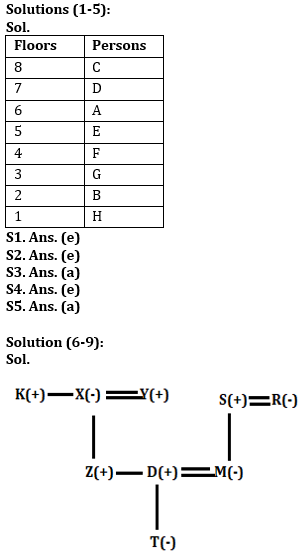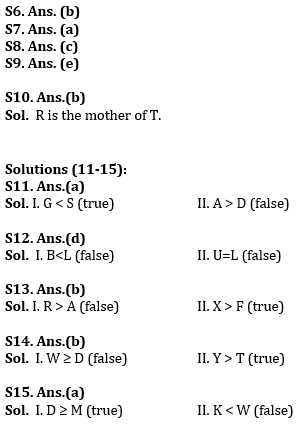Latest Banking jobs   »   Reasoning Ability Quiz For IBPS RRB...

# Reasoning Ability Quiz For IBPS RRB PO Clerk Prelims 2022- 10th July

Directions (1-5): Study the information carefully and answer the question given below.
There are eight persons A, B, C, D, E, F, G and H live in an eight-floor building (Ground floor is numbered 1 and topmost floor is number 8.) but not necessary in the same order.
F lives on floor number 4. G lives on an odd number floor below 6th floor. D lives on one of the odd number floors but not below G’s floor. There is one person living between C and A who lives just above E. B lives on the floor immediate above H. C lives on a floor above A. Not more than two persons live between A and G.

Q1. On which of the following floor G lives?
(a) 4th
(b) 5th
(c) 7th
(d) 1st
(e) 3rd

Q2. How many persons live between D and C?
(a) One
(b) Two
(c) Three
(d) Four
(e) None

Q3. If all the persons are arranged alphabetically from top to bottom then how many persons remain (live) on same floor?
(a) One
(b) Two
(c) Three
(d) Four
(e) None

Q4. Four of the following five are alike in a certain way based on their arrangement find the one which does not belong to that group?
(a) B
(b) A
(c) F
(d) C
(e) G

Q5. If C is related to A in the same way D is related to E, then following the same pattern, F is related to?
(a) B
(b) A
(c) E
(d) C
(e) None of these

Directions (6-9): Study the information carefully and answer the questions given below.
There are nine persons in three generations of family. X is the grandmother of T. D is the son in law of S. Z is the son of Y. K is the brother-in-law of Y. T is the niece of Z. R is the mother of M. T has no siblings. K is unmarried.

Q6. Who among the following person is the sister-in-law of Z?
(a) D
(b) M
(c) T
(d) X
(e) None of these

Q7. How K is related to Z?
(a) Uncle
(b) Aunt
(c) Father
(d) Grandfather
(e) None of these

Q8. If G is married to Z then who among the following is the brother in law of G?
(a) M
(b) Y
(c) D
(d) K
(e) None of these

Q9. Who among the following is the son in law of R?
(a) Z
(b) T
(c) X
(d) M
(e) None of these

Q10. R is the mother-in-law of S who is the sister-in-law of Q. P is the father of Q, who is the only brother of T. P is married to R. How is R related to T?
(a) Wife
(b) Mother
(c) Aunt
(d) Mother-in-law
(e) None of these

Directions (11-15): In these questions, relationship between different elements is shown in the statements. These statements are followed by two conclusions.
(a) If only conclusion I follows.
(b) If only conclusion II follows.
(c) If either conclusion I or II follows.
(d) If neither conclusion I nor II follows.
(e) If both conclusions I and II follow.

Q11. Statements: A≤M>G >K >D, M≤O< S, K<H
Conclusions:
I. G < S
II. A > D

Q12. Statements: B ≥ E >L<O>R=U
Conclusions:
I. B<L
II. U=L

Q13. Statements: X ≥ Q > F, A > F, R = M ≥ F
Conclusions:
I. R > A
II. X > F

Q14. Statements: D ≤ G > K ≥ R > T, G ≤ E > W, R < Y
Conclusions:
I. W ≥ D
II. Y > T

Q15. Statements: B ≥ K > G, M ≤ G, D = W ≥ G
Conclusions:
I. D ≥ M
II. K < W

Solutions#### Congratulations!Incorrect details? Fill the form again here

•Reasoning Ability Quiz For IBPS RRB PO C...
•Quantitative Aptitude Quiz For IBPS RRB ...
•Reasoning Ability Quiz For IBPS RRB PO C...
•Quantitative Aptitude Quiz For IBPS RRB ...
•Reasoning Ability Quiz For IBPS RRB PO C...
•Reasoning Ability Quiz For IBPS RRB PO C...